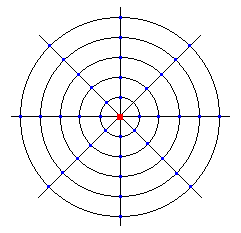# Height Above Average Terrain Worksheet

In the drawing below, the red dot is the transmitter. Each circle represents a distance of 2 miles further from the transmitter (2, 4, 6, 8 ,and 10 miles). The lines are at fixed points at 0, 45, 90, 135, 180, 225, 270, and 315 degrees. Add the ground elevations at each blue dot (where the degree lines and mileage circles intersect). Divide that number by 40 to get the average ground elevation.

Now take the transmitter's elevation and add the height of the antenna above ground to it. Now subtract the average ground elevation from it. This is the height above average terrain.Note that this can be rather misleading. It can indicate that the antenna is actually at about 150 feet when it's really only 80 or 90 feet, also if the surrounding area is higher it can even be a negative number.